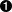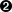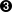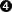Vous devez avoir un compte Developpez.com et être connecté pour pouvoir participer aux discussions.

##### Identifiez-vous
 Identifiant Mot de passe Mot de passe oublié ?
##### Créer un compte

Vous n'avez pas encore de compte Developpez.com ? L'inscription est gratuite et ne vous prendra que quelques instants !Developpez.com

Python

Choisissez la catégorie, puis la rubrique :

17.3. plural.py, stage 2

## 17.3. plural.py, stage 2

Now you're going to add a level of abstraction. You started by defining a list of rules: if this, then do that, otherwise go to the next rule. Let's temporarily complicate part of the program so you can simplify another part.

### Example 17.6. plural2.py

```
import re

def match_sxz(noun):
return re.search('[sxz]\$', noun)

def apply_sxz(noun):
return re.sub('\$', 'es', noun)

def match_h(noun):
return re.search('[^aeioudgkprt]h\$', noun)

def apply_h(noun):
return re.sub('\$', 'es', noun)

def match_y(noun):
return re.search('[^aeiou]y\$', noun)

def apply_y(noun):
return re.sub('y\$', 'ies', noun)

def match_default(noun):
return 1

def apply_default(noun):
return noun + 's'

rules = ((match_sxz, apply_sxz),
(match_h, apply_h),
(match_y, apply_y),
(match_default, apply_default)
)def plural(noun):
for matchesRule, applyRule in rules:if matchesRule(noun):return applyRule(noun)```This version looks more complicated (it's certainly longer), but it does exactly the same thing: try to match four different rules, in order, and apply the appropriate regular expression when a match is found. The difference is that each individual match and apply rule is defined in its own function, and the functions are then listed in this rules variable, which is a tuple of tuples.Using a for loop, you can pull out the match and apply rules two at a time (one match, one apply) from the rules tuple. On the first iteration of the for loop, matchesRule will get match_sxz, and applyRule will get apply_sxz. On the second iteration (assuming you get that far), matchesRule will be assigned match_h, and applyRule will be assigned apply_h.Remember that everything in Python is an object, including functions. rules contains actual functions; not names of functions, but actual functions. When they get assigned in the for loop, then matchesRule and applyRule are actual functions that you can call. So on the first iteration of the for loop, this is equivalent to calling matches_sxz(noun).On the first iteration of the for loop, this is equivalent to calling apply_sxz(noun), and so forth.

If this additional level of abstraction is confusing, try unrolling the function to see the equivalence. This for loop is equivalent to the following:

### Example 17.7. Unrolling the plural function

```
def plural(noun):
if match_sxz(noun):
return apply_sxz(noun)
if match_h(noun):
return apply_h(noun)
if match_y(noun):
return apply_y(noun)
if match_default(noun):
return apply_default(noun)
```

The benefit here is that that plural function is now simplified. It takes a list of rules, defined elsewhere, and iterates through them in a generic fashion. Get a match rule; does it match? Then call the apply rule. The rules could be defined anywhere, in any way. The plural function doesn't care.

Now, was adding this level of abstraction worth it? Well, not yet. Let's consider what it would take to add a new rule to the function. Well, in the previous example, it would require adding an if statement to the plural function. In this example, it would require adding two functions, match_foo and apply_foo, and then updating the rules list to specify where in the order the new match and apply functions should be called relative to the other rules.

This is really just a stepping stone to the next section. Let's move on.Contacter le responsable de la rubrique Python

Partenaire : Hébergement Web